# Steady State Error: What is it? (Steady-State Gain, Value & Formula)

Contents

## What is Steady State Error?

Steady-state error is defined as the difference between the desired value and the actual value of a system output in the limit as time goes to infinity (i.e. when the response of the control system has reached steady-state).

Steady-state error is a property of the input/output response for a linear system. In general, a good control system will be one that has a low steady-state error.

First, we will discuss the steady-state error in a first-order transfer function by analyzing its steady state response. Let’s consider the transfer function below: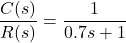This is a simple first order transfer function, having a gain equal to one and a time constant of 0.7 seconds. Note that it is known as a first-order transfer function because the ‘s’ in the denominator has the highest power of ‘1’. If it were instead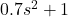, it would be a second order transfer function instead.

The response of this transfer function to a steady-state input is shown in Figure-1. It can be seen that in steady-state, the output is exactly equal to the input. Hence the steady-state error is zero.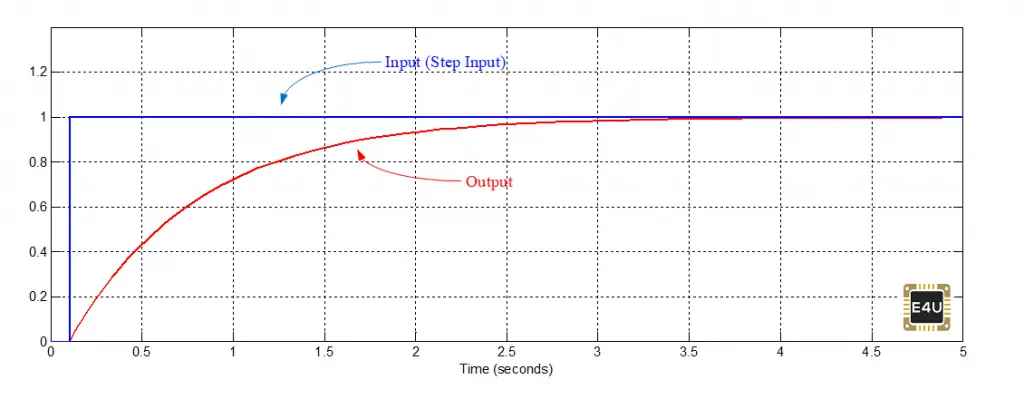Figure-1: It is the time response of First order Transfer Function against step Input. It can be seen that steady state error is zero

The response of this function to a unit ramp input is shown in Figure-2. It can be seen that in steady-state there is a difference between input and output. Hence for a unit ramp input, a steady-state error exists.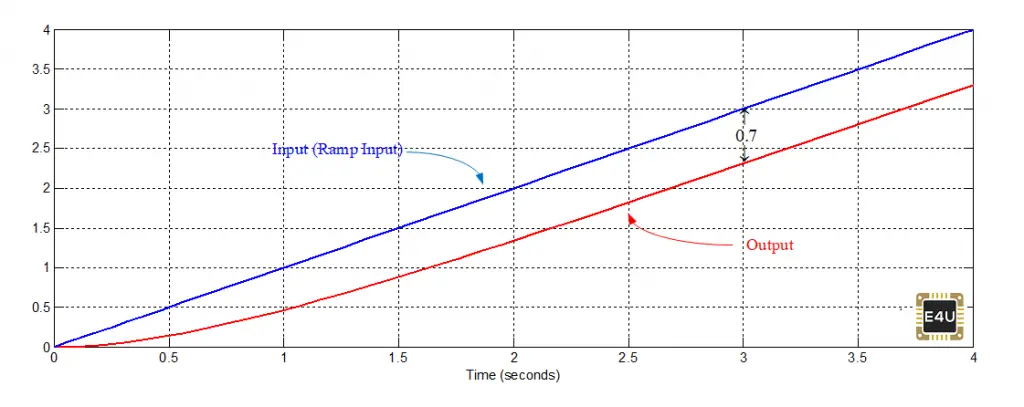Figure-2: It is the time response of First order Transfer Function against ramp Input. It can be seen that steady state error exists in this case

Note that in many control system books you can find that against ramp input, the steady-state error of a first-order transfer function is equal to the time constant. From observing Figure-2 above, we can see that this is true. At t=3 seconds, the input is 3 while the output is 2.3. Hence the steady-state error is 0.7, which is equal to the time constant for this first-order transfer function.

Please note the following important tips:

• Steady-state error is highest if the input is parabolic, is generally lower for ramp input, and is even lower for a step input. As in the above explanation, the steady-state error is zero against step input, and 0.7 against ramp input and it can be found that it is ∞ against parabolic input.
• It should be noted that steady-state error depends on input, while stability does not depend on input.

Let’s consider a closed loop control system having transfer function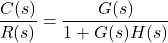Where symbols have their usual meaning. Stability of the system depends on denominator i.e. ‘1+G(s)H(s)’. ‘1+G(s)H(s) = 0’ is called characteristics equation. Its roots indicate the stability of the system. Steady-state error depends on R(s).

In a closed loop control system the error signal can be calculated as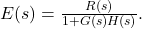Steady state error can be found as ess=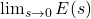, where steady-state error is the value of the error signal in steady state. From this we can see that the steady-state error depends on R(s).

• As mentioned above the stability depends on the denominator i.e. 1 + G(s)H(s). Here ‘1’ is constant, hence the stability depends on G(s)H(s), which is the part of the equation that can change. So, you can understand the Bode plot, Nyquist plot is drawn with the help of G(s)H(s), but they indicate the stability of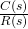.
• G(s)H(s) is called an open-loop transfer function andis called a closed-loop transfer function. By the analysis of the open-loop transfer function i.e. G(s)H(s), we can find the stability of a closed-loop transfer function through the Bode plot & Nyquist plot.

### Steady State Error for a Unit Step Input

Now, we will explain, steady-state error in a closed-loop control system with few numerical examples. We will start with a control system with a unit step input.

Example-1:

Consider the following control system (system-1) as shown in Figure-3:

Reference input ‘Rs’ is a unit step input.

Various steady-state values of System-1 are shown in Figure-4.

It can be viewed that the steady-state value of the error signal is 0.5, hence the steady-state error is 0.5. If the system is stable and various signals are constant then various steady-state values can be obtained as follows:

In the transfer function as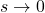, you will get the steady state gain of the transfer function.

You can calculate the output as follows: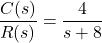Remembering that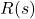= unit step input =, we can rearrange this to: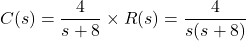The steady state value of the output is: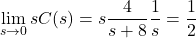We can use the above method to calculate the steady state value of any signal. For example:

Input is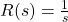(Input is unit step input)

Its steady state value=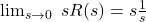= 1.

Similarly, the error signal can be calculated as: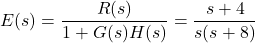The steady state value of the error signal (i.e. the steady state error) is: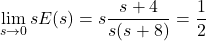Also, it can be seen from Figure-4 that the difference between the input & output is 0.5. Hence the steady state error is 0.5.

Another method to calculate steady state error involves finding the error constants, as follows:

Calculate Positional error coefficient Kp =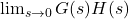, You will find Kp = 1, ess=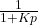. You will find the same answer.

If the input is a step input, say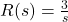(it is a step input, but not a unit step input), then steady state error is ess=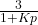If the input is unit ramp input, then Calculate, Velocity error coefficient Kv=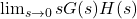, ess=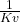If the input is unit parabolic input, then Calculate, Acceleration error coefficient Ka=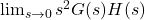, ess=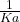.

With the analysis of the error constants Kp, Kv and Ka, you can understand how the steady state error depends on the input.

## PI Controller And Steady State Error

A PI controller (i.e. a proportional controller plus integral controller) reduces the steady state error (ess), but has a negative effect on the stability.

PI controllers have the advantage of reducing the steady-state error of a system, while having the disadvantage of reducing the system’s stability.

A PI controller reduces stability. This means that damping decreases; peak overshoot and settling time increases due to PI controller; Roots of characteristics equation (poles of closed-loop transfer function) in left-hand side will come closer to the imaginary axis. The system order also increases due to PI controller, which tends to reduce the stability.

Consider two characteristics equations, one is s3+ s2+ 3s+20=0, another is s2+3s+20=0. Just by observation, we can tell you that system related to first equation has lower stability as compared to second equation. You can verify it by finding the roots of the equation. So, you can understand higher order characteristics equations have lower stability.

Now, we will add one PI controller (Proportional Plus Integral controller) in system-1 (Figure-3) and examine the results. After inserting PI controller in system-1, various steady state values are shown in Figure-5, It can be seen that output is exactly equal to the reference input. It is the advantage of PI controller, that it minimizes the steady state error so that output tries to follow reference input.

Transfer function of PI controller can be calculated as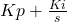or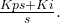One question can be asked that if the input of any transfer function is zero then its output should be zero. So, in the present case input to the PI controller is zero, but the output of the PI controller is a finite value (i.e. 1). This explanation is not given in any control system book, hence we will explain it here:

(1) Steady state error is not exactly zero, its tends to zero, similarly ‘s’ is not equal to zero, it tends to zero, So let at any instance steady state error is 2x10-3, at the same time ‘s’ (particularly we are talking about ‘s’ in the denominator of PI controller) is also equal to 2x10-3, hence output of PI controller is ‘1’.

Lets consider another control system shown in Figure-6:

In this case, we can say, at any instance suppose, steady state error is 2x10-3, at the same time ‘s’ is equal to 4×10-3; hence the output of PI controller is ‘0.5’. It means both ‘ess ‘ and ‘s’ both tend to zero, but their ratio is a finite value.

In the books of control system you will never find s=0 or t=∞; you will always find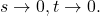(2) The second explanation is that steady-state error is zero, ‘s’ is also zero in steady state. PI controller transfer function is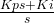.  In the books of mathematics, you will find thatis undefined, so it can be any finite value (refer Figure-7).

(3) Third explanation is,is an integrator. Input is zero, integration of zero is undefined. So the output of the PI controller may be any finite value.

One basic difference in open loop control system & closed loop control system

In reference to the above explanation, we will explain one basic difference in an open-loop control system & a closed-loop control system. Differences in open-loop control system & closed-loop control system, you can find in any book of control systems*, but one basic difference which is related to the above explanation is given here and we hope certainly it will be useful for the readers.

An open loop control system can be represented as follows:

A closed loop control system (feedback control system) can be represented as follows:

The transfer function of the plant is fixed (Transfer Function of the plant can be changed automatically due to environmental change, disturbances etc.). In all our discussion, we have assumed H(s)=1; An operator can control the transfer function of the controller (i.e parameter of the controller such that Kp, Kd, Ki) etc.

The controller can be Proportional controller (P controller), PI controller, PD controller, PID controller, Fuzzy logic controller etc. There are two aims of a controller (i) To maintain stability, i.e. damping should be around 0.7-0.9, peak overshoot and settling time should be low (ii) Steady-state error should be minimum (it should be zero).

But if we will try to increase the damping then the steady-state error may increase. Therefore designing the controller should be such that both (stability & steady-state error) should be within control. The optimal design of the controller is a vast research topic.

It is written earlier, PI controller reduces the Steady state error (ess) drastically, but have negative effect on the stability.

Now, we will explain one basic difference between open loop control system & closed loop control system, which is related to above explanation.

Consider Figure-10; it is an open loop control system.

Let input is a unit step input. So, the steady-state value of input is ‘1’. It can be calculated that steady state value of output is ‘2’. Suppose there is a change in transfer function [G(s)] of the plant due to any reason, what will be the effect on input & output? The answer is the input to the plant will not change, output of the plant will change.

Now consider Figures-11 &12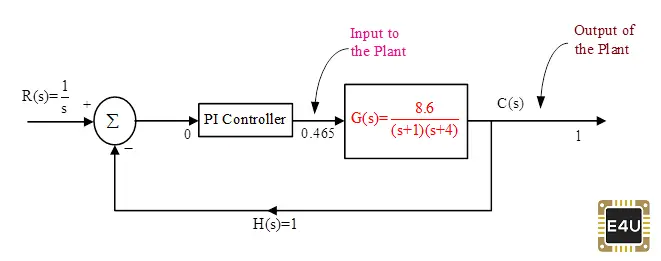Figure-12: Closed Loop System, Plant output is same but plant input is changed due to change in Transfer Function

Both are closed loop control systems. In Figure-11, suppose there is a change in the transfer function of the plant due to any reason, what will be the effect on input & output? In this case, input to the plant will change, output of the plant will remain unchanged. Output of the plant tries to follow reference input.

Figure -12 shows the new conditions, in which plant parameters is changed. You can see plant input is changed to 0.476 from 0.5, while the output is not changed. In both the cases input to the PI controller is zero, the specifications of the PI controller are the same but the output of the PI controller is different.

So, you can understand, in the open loop control system output of the plant is changed while in closed loop control system input to the plant is changed.

In the books of the control system, you can find the following statement:

“In case of parameter variation of plant transfer function, closed loop control system is less sensitive as compared to open loop control system” (i.e. variation in output of closed loop control system is less as compared to open loop control system).

We hope above statement may be more clear with the examples given in this article.

___________________________________________________________________